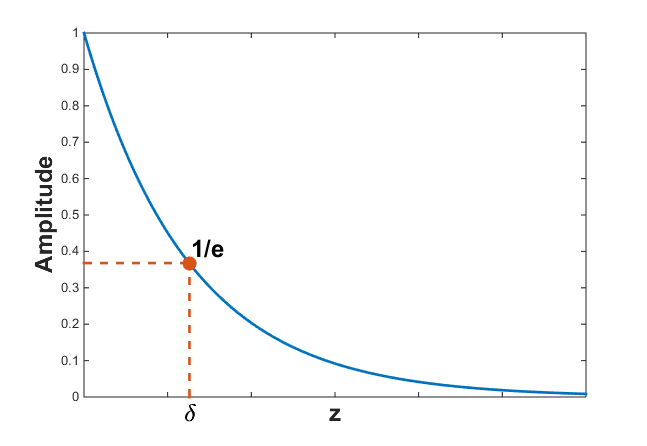# Attenuation and Skin DepthFig. 45 Skindepth is defined as the depth at which the amplitude of the wave has been reduced by $$1/e$$.

## Attenuation

Attenuation defines the rate of amplitude loss an EM wave experiences at it propagates (Fig. 45). The attenuation of an EM wave is defined by the parameter $$\beta$$. For a downgoing planewave, the attenuation formula is given by:

$A(z) = A_0 e^{\beta z}$

where absolute $$A$$ is the amplitude, $$A_0$$ is the absolute amplitude at $$z$$ = 0 m and:

$\beta = \omega \left ( \frac{\mu\epsilon}{2} \left [ \left ( 1 + \frac{\sigma^2}{\epsilon^2 \omega^2} \right)^{1/2} - 1 \right ] \right ) ^{1/2} \geq 0$

## Skin Depth

Skin depth defines the distance a wave must travel before its amplitude has decayed by a factor of $$1/e$$. The skin depth is the reciprocal of the decay constant $$\beta$$. Thus:

$\delta = \frac{1}{\beta} = \frac{1}{\omega} \left ( \frac{\mu\epsilon}{2} \left [ \left ( 1 + \frac{\sigma^2}{\epsilon^2 \omega^2} \right)^{1/2} - 1 \right ] \right ) ^{1/2}$

Since $$\beta$$ depends on the frequency and the physical properties of the media, so does the skin depth. For a general case, the skin depth can be considered a fairly complicated function. However, approxmations exist in the quasi-static and wave regimes.

### Skin Depth for Various Materials

The table below shows skin depths for certain rocks at various frequencies. This is meant to serve as a general guide, as rock types are classified by a range of physical properties values which can lead to order of magnitude differences in skin depth.

Type

$$\sigma$$

$$\mu_r$$

$$\epsilon_r$$

$$\delta$$ (1Hz)

$$\delta$$ (1kHz)

$$\delta$$ (1MHz)

$$\delta$$ (1GHz)

Air

0 S/m

1

1

$$\infty$$

$$\infty$$

$$\infty$$

$$\infty$$

Sea Water

3.3 S/m

1

80

277 m

8.76 m

0.277 m

0.015 m

Igneous

$$10^{-4}$$ S/m

1

5

50,300 m

1,590 m

121 m

119 m

Sedimentary (dry)

$$10^{-3}$$ S/m

1

4

15,900 m

500 m

18 m

11 m

Sedimentary (wet)

$$10^{-2}$$ S/m

1

25

5,000 m

160 m

5.4 m

2.6 m

Sulphide Skarn

$$10^{2}$$ S/m

1

5

50 m

1.6 m

0.05 m

0.002 m

Magnetite Skarn

$$10^{2}$$ S/m

2

5

36 m

1.1 m

0.04 m

0.001 m

### Approximations

Quasi-Static Approximation

In the quasi-static regime ($$\epsilon\omega \ll \sigma$$), the skin depth is approximately equal to:

$\delta = \frac{1}{\beta} = \sqrt{\frac{2}{\omega \mu \sigma}}.$

Assuming the Earth is non-magnetic ($$\mu = \mu_0 = 4\pi \times 10^{-7}$$ H/m) and replacing $$\omega=2\pi f$$, a simpler form of the skin depth is given by:

$\delta \approx 503 \sqrt{\frac{1}{f \sigma}} = 503 \sqrt{\frac{\rho}{f}}.$

where $$\rho = 1/\sigma$$ is the resistivity. Thus from the previous two equations, we see that the skin depth decreases as the conductivity $$\sigma$$, magnetic permeability $$\mu$$ and frequency $$\omega$$ increase. In most cases however, the magnetic properties are negligible as $$\mu \sim \mu_0$$.

Wave-Regime Approximation

In the wave regime ($$\epsilon\omega \gg \sigma$$), the skin depth is approximately equal to:

$\delta = \frac{1}{\beta} = \frac{2}{\sigma} \sqrt{\frac{\epsilon}{\mu}}$

Assuming the Earth is non-magnetic ($$\mu = \mu_0 = 4\pi \times 10^{-7}$$ H/m) and by using the relative permittivity $$\epsilon_r = \epsilon/\epsilon_0$$, a simpler form of the skin depth is given by:

$\delta \approx 0.0053 \frac{\sqrt{\epsilon_r}}{\sigma}$

Thus from the previous two equations, we see that the skin depth decreases proportional $$\sqrt{\epsilon_r}/\sigma$$; provided the magnetic properties are negligible ($$\mu \sim \mu_0$$). The below shows skin depths for some representative rocks and frequencies in the wave regime. To see how physical properties and frequencies affect skin depth, check out the app.